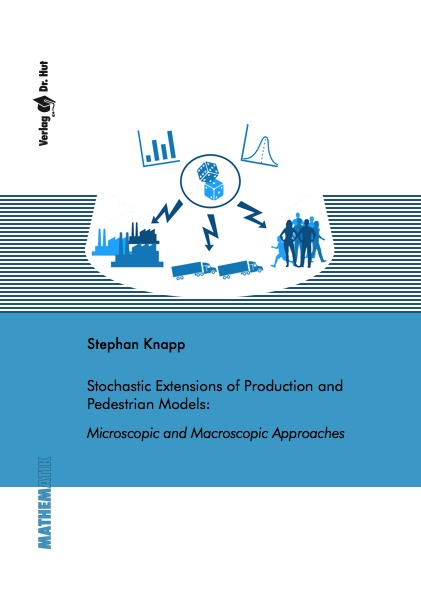Datenbestand vom 15. Oktober 2021aktualisiert am 15. Oktober 2021

# ISBN 978384393691084,00 € inkl. MwSt, zzgl. Versand

978-3-8439-3691-0, Reihe Mathematik

Stephan Knapp
Stochastic Extensions of Production and Pedestrian Models: Microscopic and Macroscopic Approaches

253 Seiten, Dissertation Universität Mannheim (2018), Softcover, A5

## Zusammenfassung / Abstract

We investigate and extend two types of mathematical models, production and interacting particle models, in this thesis. The main focus is on fusing the theory of deterministic models based on ordinary and partial differential equations, respectively, with the theory of stochastic processes. Additionally, we develop appropriate simulation schemes and apply them to various examples, subsequently studying the characteristics of the models numerically.

The first part of this work is related to production models. Starting from a deterministic production network model, we extend this model with capacities based on semi-Markov processes in a first step. We study the impact of different capacity states and various distributions of the time between capacity drops intensively and compare the model to a well-established queueing system. In a second step, we derive a production network model that allows for a bidirectional relation between random capacity drops and the workload of the production. We study and validate the new model dynamics in different scenarios. For both models, we use and compare classical evaluations, such as the expected value and variance, with risk measures taken from the field of mathematical finance. Based on the link between queueing and production models, we develop a continuous approximation model for a time-dependent Markovian queueing system and apply it to the production setting.

The thesis’s second part contains stochastic extensions and macroscopic limits of a pedestrian and aggregation-diffusion model. Concerning the pedestrian model, we derive an appropriate closure distribution to obtain a first order macroscopic model. In the aggregation-diffusion model, we state theoretical results, starting from the stochastic microscopic system to the limiting equation. We thoroughly study the threshold from aggregation to diffusion (and vice versa) numerically with problem-adapted numerical schemes. For both models, the pedestrian and aggregation-diffusion model, we study the convergence of the microscopic description to the macroscopic equations numerically and validate the models by various examples.